Select Page

# CBSE MCQ in English Answers for Maths 12 Science Inverse Trigonometric Functions

CBSE MCQ in English Answers for Maths 12 Science Inverse Trigonometric Functions to enable students to get Answers in a narrative video format for the specific question.

Expert Teacher provides CBSE MCQ Answers for Maths 12 Science Inverse Trigonometric Functions through Video Answers in English language. This video solution will be useful for students to understand how to write an answer in exam in order to score more marks. This teacher uses a narrative style for a question from Inverse Trigonometric Functions not only to explain the proper method of answering question, but deriving right answer too.

Please find the question below and view the Answer in a narrative video format.

Question:

## Similar Questions from CBSE, 12th Science, Maths, Inverse Trigonometric Functions

Question 1 : Solve for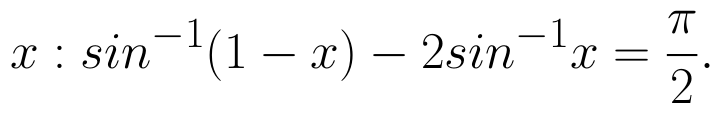(View Answer Video)

Question 2 : Evaluate :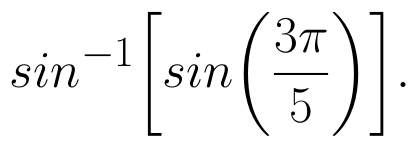(View Answer Video)

Question 3 :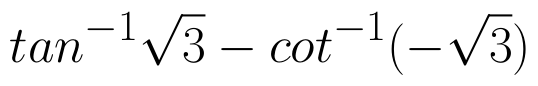is equal to : (View Answer Video)

Question 4 : Solve the equation for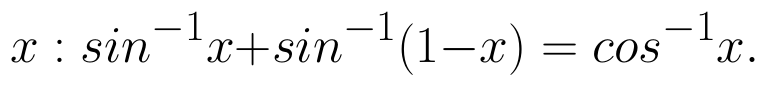(View Answer Video)

Question 5 : If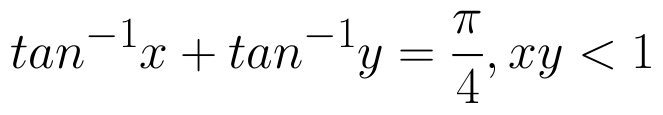, then write the value of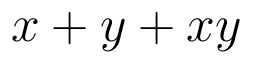. (View Answer Video)

### Determinant

Question 1 : Let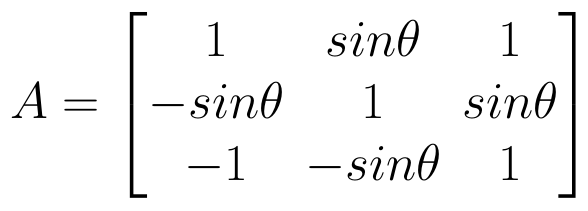, where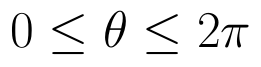. THhen (View Answer Video)

Question 2 : Let A be the non- singular square matrix of order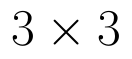, then |adj A| is equal to, (View Answer Video)

Question 3 : Using the properties of determinants, evaluate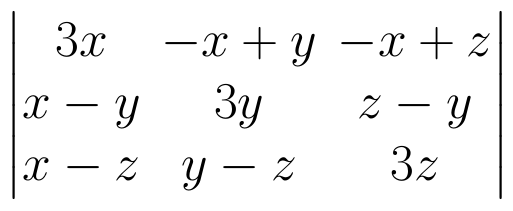. (View Answer Video)

Question 4 : Find the inverse of the matrix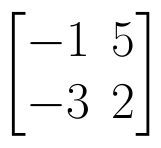. (View Answer Video)

Question 5 : If A =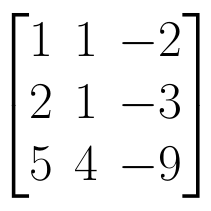, find | A |. (View Answer Video)

### Application of Derivatives

Question 1 : The total revenue (in Rs) received from the sale of 'x' units of a product is given by :
R(x) =3x2+36x+5.
FInd the marginal revenue when x=5, where by marginal revenue we mean the rate of change of total revenue with respect to the number of items sold at an instant. (View Answer Video)

Question 2 : The line y=mx+1 is a tangent to the curve if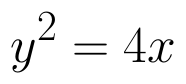the value of m is ________. (View Answer Video)

Question 3 : The line y = mx + 1 is a tangent to the curve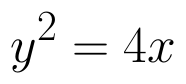if the value of m is: (View Answer Video)

Question 4 : The normal to the curve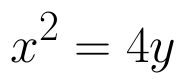passing (1, 2) is : (View Answer Video)

Question 5 : The line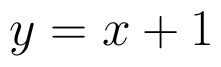is a tangent to the curveat the point. (View Answer Video)

### Relations and Functions

Question 1 :  A function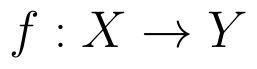is surjective if and only if , (View Answer Video)